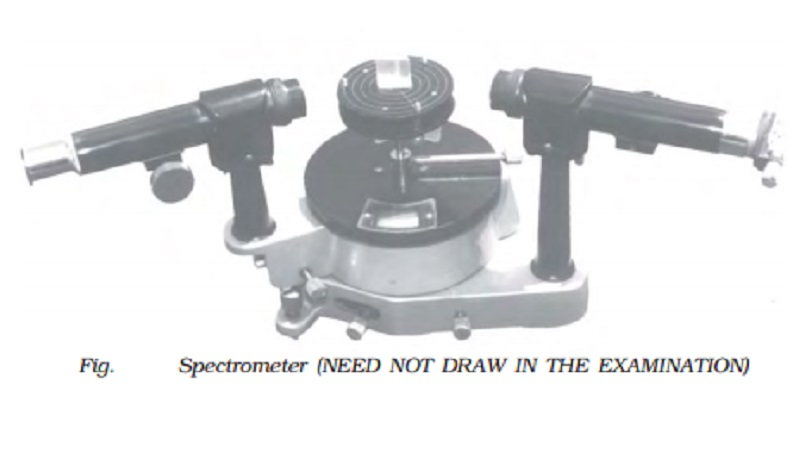Home | | Physics | | Physics | Spectrometer

# SpectrometerThe spectrometer is an optical instrument used to study the spectra of different sources of light and to measure the refractive indices of materials (Fig. ). It consists of basically three parts. They are collimator, prism table and Telescope.

Spectrometer

The spectrometer is an optical instrument used to study the spectra of different sources of light and to measure the refractive indices of materials (Fig. ). It consists of basically three parts. They are collimator, prism table and Telescope.Collimator

The collimator is an arrangement to produce a parallel beam of light. It consists of a long cylindrical tube with a convex lens at the inner end and a vertical slit at the outer end of the tube. The distance between the slit and the lens can be adjusted such that the slit is at the focus of the lens. The slit is kept facing the source of light. The width of the slit can be adjusted. The collimator is rigidly fixed to the base of the instrument.

Prism table

The prism table is used for mounting the prism, grating etc. It consists of two circular metal discs provided with three levelling screws. It can be rotated about a vertical axis passing through its centre and its position can be read with verniers V1 and V2. The prism table can be raised or lowered and can be fixed at any desired height.

Telescope

The telescope is an astronomical type. It consists of an eyepiece provided with cross wires at one end of the tube and an objective lens at its other end co-axially. The distance between the objective lens and the eyepiece can be adjusted so that the telescope forms a clear image at the cross wires, when a parallel beam from the collimator is incident on it.

The telescope is attached to an arm which is capable of rotation about the same vertical axis as the prism table. A circular scale graduated in half degree is attached to it.

Both the telescope and prism table are provided with radial screws for fixing them in a desired position and tangential screws for fine adjustments.

The following adjustments must be made before doing the experiment with spectrometer.

The telescope is turned towards an illuminated surface and the eyepiece is moved to and fro until the cross wires are clearly seen.

The telescope is adjusted to receive parallel rays by turning it towards a distant object and adjusting the distance between the objective lens and the eyepiece to get a clear image on the cross wire.

The telescope is brought along the axial line with the collimator. The slit of the collimator is illuminated by a source of light. The distance between the slit and the lens of the collimator is adjusted until a clear image of the slit is seen at the cross wires of the telescope. Since the telescope is already adjusted for parallel rays, a well defined image of the slit can be formed, only when the light rays emerging from the collimator are parallel.

(iv) Levelling the prism table

The prism table is adjusted or levelled to be in horizontal position by means of levelling screws and a spirit level.

Determination of the refractive index of the material of the prism

The preliminary adjustments of the telescope, collimator and the prism table of the spectrometer are made. The refractive index of the prism can be determined by knowing the angle of the prism and the angle of minimum deviation.

(i) Angle of the prism (A)

The prism is placed on the prism table with its refracting edge facing the collimator as shown in Fig . The slit is illuminated by a sodium vapour lamp.The parallel rays coming from the collimator fall on the two faces AB and AC.

The telescope is rotated to the position T1  until the image of the slit, formed by the reflection at the face AB is made to coincide

with the vertical cross wire of the telescope. The readings of the verniers are noted. The telescope is then rotated to the position T2  where the image of the slit formed by the reflection at the face AC coincides with the vertical cross wire. The readings are again noted.

The difference between these two readings gives the angle rotated by the telescope. This angle is equal to twice the angle of the prism. Half of this value gives the angle of the prism A.

(ii) Angle of minimum deviation (D)

The prism is placed on the prism table so that the light from the collimator falls on a refracting face, and the refracted image is observed through the telescope (Fig. ). The prism table is now rotated so that the angle of deviation decreases. A stage comes when the image stops for a moment and if we rotate the prism table further in the same direction, the image is seen to recede and the angle of deviation increases. The vertical cross wire of the telescope is made to coincide with the image of the slit where it turns back. This gives the minimum deviation position. The readings of the verniers are noted. Now the prism is removed and the telescope is turned to receive the direct ray and the vertical cross wire is made to coincide with the image. The readings of the verniers are noted. The difference between the two readings gives the angle of minimum deviation D.

The refractive index of the material of the prism ? is calculated using the formula ? = sin((A+D) / 2) / sin(A/2)The refractive index of a liquid may be determined in the same way using a hollow glass prism filled with the given liquid.

Study Material, Lecturing Notes, Assignment, Reference, Wiki description explanation, brief detail

Related Topics